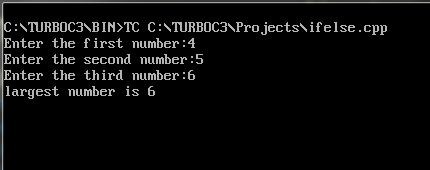UncleCoder.com

UncleCoder.com

Free programming examples and instructions

# CPP Program to study if control statement

## CPP Program to study how to use if control statement

by Krishna

Posted on 27 Jun 2018 Category: c-plus-plus Views: 931

CPP Program to study if control statement

In this article we will discuss about the basic CPP control structures and its implementation. Like C programming language, CPP also supports all basic control structures such as if, if..else, switch, while, do..while, for loop. So here we will discuss in detail about the ‘if control statement’. Syntax is same as the C if statement.

The if statement is implemented in two forms

• Simple if statement
``````if(true condition)
{
Action;
}
``````

• if…else statement
``````if(true condition)
{
Action;
}
else
{
Action;
}
``````

Here is a program which demonstrates the if control statement. This program calculates the largest number among the three given numbers.

``````#include<iostream>
#include<conio.h>
using namespace std;
void main()
{
int num1, num2, num3;
cout<<"Enter the first number ";
cin>>num1;
cout<<"Enter the second number: ";
cin>>num2;
cout<<"Enter the third number: ";
cin>>num3;
if((a>b)&&(a>c))
{
cout<< “ largest number is”<<num1;
}
else if(b>c)
{
cout<< “largest number is”<<num2;
}
else
{
cout<< “largest number is”<<num3;
}
getch();
}
``````

In the above program, the first if statement checks whether the first number is greater than the second and third number, if it is true action is executed(here, prints the largest number) and if it is false, the control goes to the if else part or else  part.

C code for the above program is also available in unclecoder.com

Output for the above program is shown as:

OUTPUT